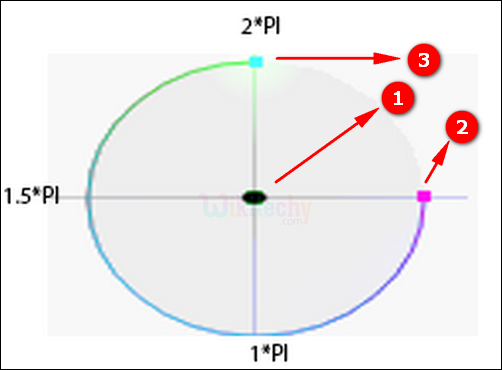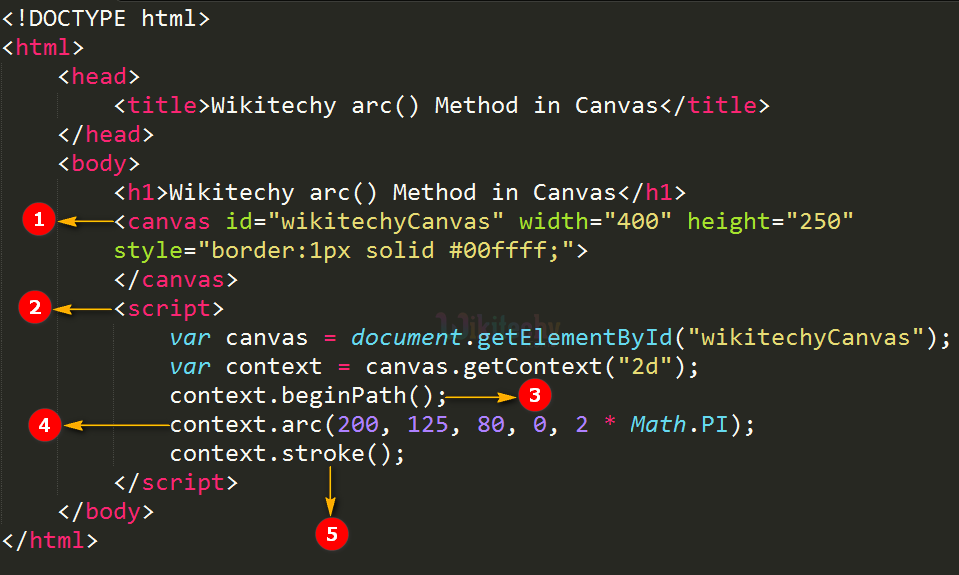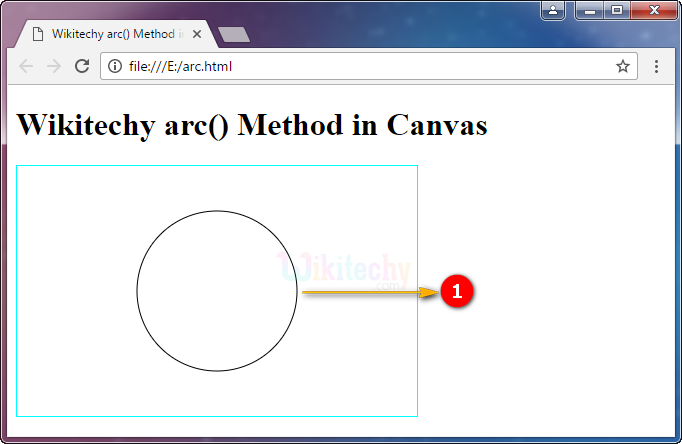# html tutorial - arc() Method in HTML5 Canvas - html5 - html code - html formLearn html - html tutorial - Arc method in html5 canvas - html examples - html programs

• arc() Method is used to create a arc or curve
• It is used to create circles, or parts of circles.
•• Center arc (100, 75, 50, 0*Math.PI, 1.5*Math.PI)
• Start angle arc (100, 75, 50, 0, 1.5*Math.PI)
• End angle arc (100, 75, 50, 0*Math.PI, 1.5*Math.PI)

## Parameter values for arc() Method in HTML5 Canvas:

Parameter Description
X The x-coordinate of the center of the circle
Y The y-coordinate of the center of the circle
sAngle The starting angle, in radians.
eAngle The ending angle, in radians.
counterclockwise It denotes whether the drawing should be Anticlockwise or clockwise. This is an optional value.

## Code Explanation for arc() Method in HTML5 Canvas:1. The <canvas> tag is used to draw a rectangle with width=”400” and height=”250”.
2. The <script> tag is used to draw a two dimensional circle in the canvas.
3. The beginPath() method is used to begins a path, or resets the current path.
4. arc() is used to create circle on canvas(200, 125, 80, 0, 2 * Math.PI);
5. The stroke() method is used to draw the path.

## Output for arc() Method in HTML5 Canvas:1. Here the output displays circle with radius 80.

## Browser Support for arc() Method in HTML5 Canvas:Yes 9.0 Yes Yes Yes